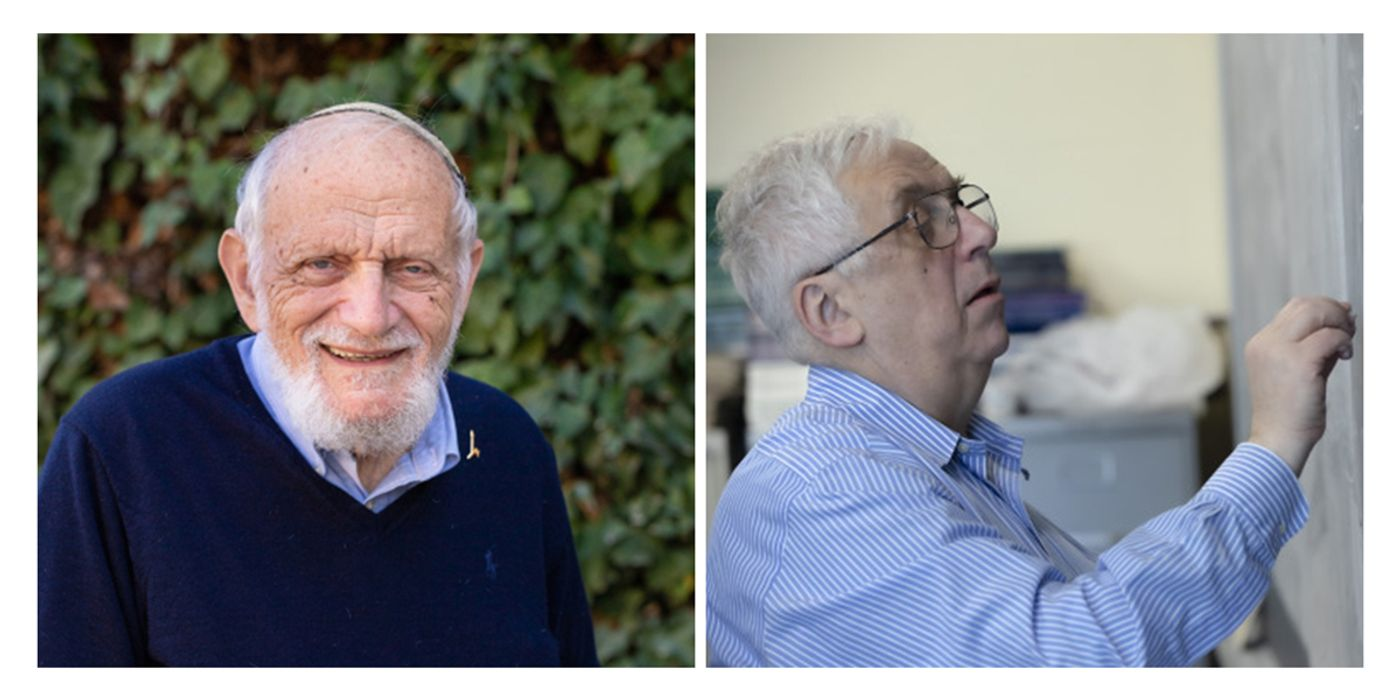MAR 21, 2020 6:17 AM PDT

# Ergodic Theory Earned Retired Duos the Top Prize in MathsThe Abel Prize, organized by the Norwegian Academy of Science and Letters, is the world's highest honor in mathematics. It was established in memory of Norwegian mathematician Niels Henrik Abel and handed out its first award in 2003.

Earlier this week, the prize committee announced that the 2020 laureates are two retired professors, Hillel Furstenberg at the Hebrew University of Jerusalem, and Gregory Margulis at Yale University. The duo is honored "for pioneering the use of methods from probability and dynamics in group theory, number theory, and combinatorics".

Both Furstenberg and Margulis made a tremendous contribution to a specific area in arithmetics: ergodic theory. This branch of mathematics examines the time-dependent randomness of an ever-changing system that's yet mathematically solvable.

Ergodic theory was developed to study complex, chaotic behaviors in nature, such as the planetary system and weather pattern, problems that can be better understood with statistical physics.

American mathematician Edward Lorenz pioneered the chaos theory when he was working on weather simulation back in the 1960s. Using a computer-run simulation program, he discovered that any slightest change of input parameters could lead to a dramatically different outcome. His mathematical investigation led to the birth of chaos theory, which states that the degree of chaos in a complex system becomes magnified even when a tiny change is introduced.

The two mathematicians were the first to use random walk techniques in mathematical studies. A random walk technique, as its name indicates, describes a mathematical path that is made of a succession of random steps. The hypothesis that bears the same name is now a popular tool in financial sectors, due to its capability to model market and economic patterns. By applying random walk models, the duo developed valuable, probability-based methods to solve problems in multiple mathematical theories.

The two retired professors will share the cash award worth about 834,000 U.S. dollars. Last year, the Abel prize committee picked their first female laureate, Karen Keskulla Uhlenbeck, a professor emeritus from the University of Texas at Austin and a pioneer in geometry and mathematical physics.

Interested in learning more about chaos theory? Check out the following Youtube video from Seeker.

How Chaos Theory Unravels the Mysteries of Nature (Seeker)

Source: Nature News